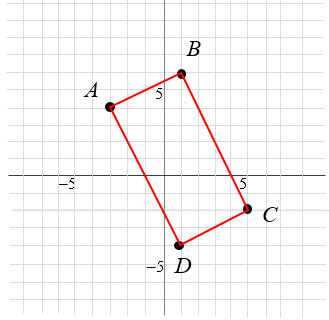### Home > INT2 > Chapter 2 > Lesson 2.1.1 > Problem2-8

2-8.

Plot the following points on a sheet of graph paper and connect them in the order given. Then connect points $A$ and $D$.

$A(−3, 4), B(1, 6), C(5, −2), \operatorname{and} D(1, −4)$

1. A rectangle is a four-sided polygon with four right angles. Does the shape you graphed appear to be a rectangle? Use slope to justify your answer.

Use the graph to help find the slopes. How do the slopes of perpendicular lines compare?2. If $ABCD$ is rotated $90^\circ$ clockwise ($\circlearrowright$) about the origin to form $A′B′C′D′$, what are the coordinates of the vertices of $A′B′C′D′$?

Since the origin is at $(0,0),$ rotate each point in correlation to this point.

$A'(4,3),B'(6,−1),C'(−2,−5),−2,−5),D'(−4,−1)$.